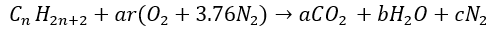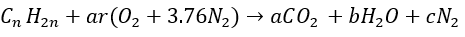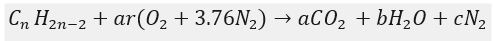## Plot & Calc. the stoichiometric coefficient for diff. fuels

Versión 1.0.0 (1.41 KB) por
Calculation the stoichiometric coefficient for diff. fuels and simulation various plots depicting the effect of no. of moles and Sto.eff.

34 descargas

Ver licencia

We have been given a standard reaction which depicts the formation of oxygen, nitrogen and hydrogen by burning if fuel, which is as follows,Here,
ar = stoichiometric coefficient
a = number of moles of carbon-dioxide
b = number of moles of water
c = number of moles of nitrogen
Methodology:
• Theory behind combustion
Since ages combustion is been regarded as one of the most essential processes in production of fuel. Combustion is a method or a reaction where the fuel is burnt in presence of oxygen and the products are generally in the form of a smoke. When combustion takes place industrially, the oxidizer that oxidizes the fuel is air.
In terms of calculation, dry air contains 20.96% oxygen, 78.21% nitrogen and the remaining gases (CO2, argon, neon and some gases of trace amounts) are left for other inert gases to occupy. While the combustion is taking place inside an industry, all the inert gases are neglected and only oxygen and nitrogen are taken into consideration. So, if we go by mass balance of both the gases, result for division of 0.79 (79% N2) and 0.21 (21% O2) will give us with a factor of 3.76. From that factor, we can rewrite for production of air as,
From our earlier elementary knowledge on basic chemistry, we know that each and every reaction that takes place on earth has to be mass balanced by the law of conservation of mass, respectively. Here, we balance the mass and moles of for elements of the given reaction in order to get the stoichiometric coefficient for alkanes, alkenes and alkynes type fuels.
Please note that, after theoretically calculating the stoichiometric coefficients for alkanes, alkenes and alkynes, we then code the same process in MATLAB in order to plot and find out the effect of number of moles and stoichiometric coefficients for different gases and state their results in conclusion.
• Calculation of stoichiometric coefficients for different gases:
• For alkanes,
We have,Here, after applying mass balance for the above equation we get,
For Carbonn = a
For Hydrogen – 2n+ 2 = 2b -› n + 1 = b
For Oxygen – 2 * ar = 2a + b
For Nitrogen – 3.76 * 2 * ar = 2c
So, therefore, we have to find “ar” in terms of “n”,
2ar = 2n + n + 1
ar = – (1)
similarly,
• For alkenes,
We have,Here, after applying mass balance for the above equation we get,
For Carbonn = a
For Hydrogen – 2n= 2b -› n = b
For Oxygen – 2 * ar = 2a + b
For Nitrogen – 3.76 * 2 * ar = 2c
So, therefore, we have to find “ar” in terms of “n”,
2ar = 2n + n
ar = 3n – (2)
Finally,
• For alkynes,
We have,Here, after applying mass balance for the above equation we get,
For Carbonn = a
For Hydrogen – 2n - 2 = 2b -› n 1 = b
For Oxygen – 2 * ar = 2a + b
For Nitrogen – 3.76 * 2 * ar = 2c
So, therefore, we have to find “ar” in terms of “n”,
2ar = 2n + n – 1
ar = – (2)
After, finally calculating all the stoichiometric coefficients, we write the MATLAB code and observe the result.
Conclusion:
1. We can see that all the three graphs are linear which means that the effect of stoichiometric coefficients with respect to all the three gases (fuels) are more or less the same.
2. The y-intercept for alkynes is the lowest as compared to that of alkanes and alkenes because the no. of moles of hydrogen is more which could curtail the rate of combustion thereby resulting into a low fuel-efficiency. Whereas, the combustion efficiency of alkynes is higher as the number of hydrogen atoms are smaller as compared to that of oxygen and more of fuel is burned thereby resulting into a greater number of oxidized products, respectively.
3. More the number of moles of oxygen, better will the fuel burn and this regards to vice-versa for number of moles of hydrogen when oxidation of fuel is taken into consideration.

### Citar como

Iyer Aditya Ramesh (2023). Plot & Calc. the stoichiometric coefficient for diff. fuels (https://www.mathworks.com/matlabcentral/fileexchange/94175-plot-calc-the-stoichiometric-coefficient-for-diff-fuels), MATLAB Central File Exchange. Recuperado .

##### Compatibilidad con la versión de MATLAB
Se creó con R2021a
Compatible con cualquier versión